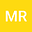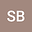••In this work, we give an exact solution to nonlinear system of coupled time-fractional Boussinesq-Burger equation using the Fractional Natural Decomposition Method (FNDM). Also, we find approximate solutions to nonlinear fractional system of ordinary differential equations and exact solutions in the case when $\alpha=\beta=\gamma=1$. The numerical computations in the tables show that our analytical solutions converge very rapidly to the exact solutions